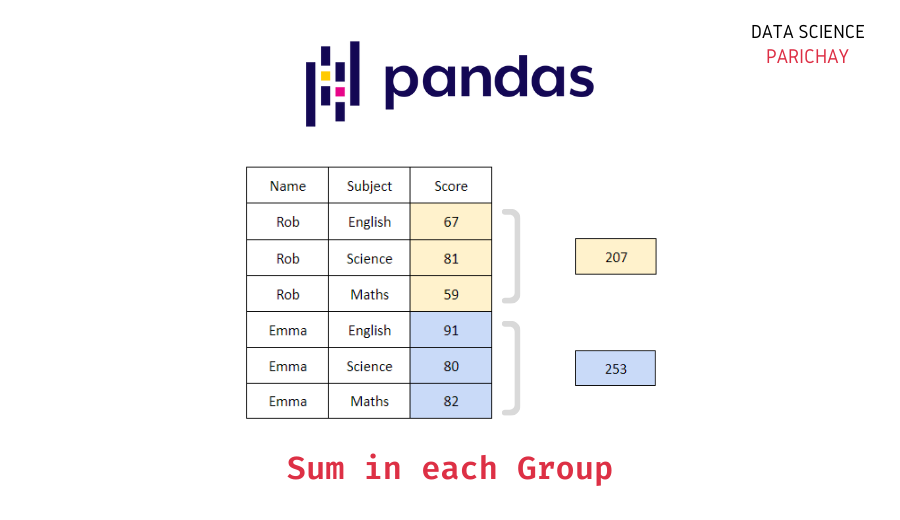# Get Sum for Each Group in Pandas Groupby

You can use Pandas groupby to group the underlying data on one or more columns and estimate useful statistics like count, sum, mean, median, min, max etc. In this tutorial, we will look at how to get the sum for each group in pandas groupby with the help of some examples.

To get the sum (or total) of each group, you can directly apply the pandas `sum()` function to the selected columns from the result of pandas groupby. The following is a step-by-step guide of what you need to do.

1. Group the dataframe on the column(s) you want.
2. Select the field(s) for which you want to estimate the sum.
3. Apply the pandas `sum()` function directly or pass ‘sum’ to the `agg()` function.

The following is the syntax –

```# groupby columns Col1 and estimate the sum of column Col2
df.groupby([Col1])[Col2].sum()
# alternatively, you can pass 'sum' to the agg() function
df.groupby([Col1])[Col2].agg('sum')```

Let’s look at some examples of using the above syntax to get the sum of each group in pandas groupby. First, we will create a sample dataframe that we will be using throughout this tutorial.

```import pandas as pd

# create a dataframe of car models by two companies
df = pd.DataFrame({
'Name': ['Rob', 'Rob', 'Rob', 'Emma', 'Emma', 'Emma', 'Hasan', 'Hasan', 'Hasan'],
'Subject': ['English', 'Science', 'Maths', 'English', 'Science', 'Maths', 'English', 'Science', 'Maths'],
'Marks': [67, 81, 59, 91, 80, 82, 73, 76, 54],
'Projects': [0, 1, 0, 1, 1, 0, 0, 2, 0]
})
# display the dataframe
df```

Output:

Here we created a dataframe storing the marks obtained by some students in a high school in different subjects. The “Projects” field tells the number of projects submitted by the student in that subject.

Let’s compute the total marks obtained by each student in the dataframe. For this, we need to group the dataframe df on “Name” and then calculate the sum of the “Marks” column.

```# total marks for each student
df.groupby('Name')['Marks'].sum()```

Output:

📚 Data Science Programs By Skill Level

Introductory

Intermediate ⭐⭐⭐

🔎 Find Data Science Programs 👨‍💻 111,889 already enrolled

Disclaimer: Data Science Parichay is reader supported. When you purchase a course through a link on this site, we may earn a small commission at no additional cost to you. Earned commissions help support this website and its team of writers.

```Name
Emma     253
Hasan    203
Rob      207
Name: Marks, dtype: int64```

You can see that we get the total marks obtained by each student. “Emma” scored the most marks considering all the subjects among the three students.

Alternatively, you can also use the `agg()` function with “sum” as the argument to estimate the sum of each group in pandas groupby.

```# total marks for each student
df.groupby('Name')['Marks'].agg('sum')```

Output:

```Name
Emma     253
Hasan    203
Rob      207
Name: Marks, dtype: int64```

We get the same result as above.

You can also get the sum of multiple columns at a time for each group. For example, let’s get the sum of marks and project submissions for each student.

```# total marks and project submissions for each student
df.groupby('Name')[['Marks', 'Projects']].sum()```

Output:

Here, we grouped the data on “Name” and then calculated the sum of the values in columns “Marks” and “Projects” for each group.

Alternatively, we can use the `agg()` function with “sum” as the argument.

```# total marks and project submissions for each student
df.groupby('Name')[['Marks', 'Projects']].agg('sum')```

Output:

We get the same results as above.

With this, we come to the end of this tutorial. The code examples and results presented in this tutorial have been implemented in a Jupyter Notebook with a python (version 3.8.3) kernel having pandas version 1.0.5

•